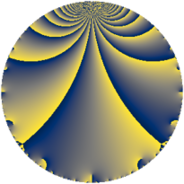Properties

 Label 1800.2.k.eLevel $1800$ Weight $2$ Character orbit 1800.k Analytic conductor $14.373$ Analytic rank $1$ Dimension $2$ CM discriminant -24 Inner twists $4$

Related objects

Newspace parameters

 Level: $$N$$ $$=$$ $$1800 = 2^{3} \cdot 3^{2} \cdot 5^{2}$$ Weight: $$k$$ $$=$$ $$2$$ Character orbit: $$[\chi]$$ $$=$$ 1800.k (of order $$2$$, degree $$1$$, minimal)

Newform invariants

 Self dual: no Analytic conductor: $$14.3730723638$$ Analytic rank: $$1$$ Dimension: $$2$$ Coefficient field: $$\Q(\sqrt{-2})$$ Defining polynomial: $$x^{2} + 2$$ Coefficient ring: $$\Z[a_1, a_2]$$ Coefficient ring index: $$1$$ Twist minimal: no (minimal twist has level 72) Sato-Tate group: $\mathrm{U}(1)[D_{2}]$

$q$-expansion

Coefficients of the $$q$$-expansion are expressed in terms of $$\beta = \sqrt{-2}$$. We also show the integral $$q$$-expansion of the trace form.

 $$f(q)$$ $$=$$ $$q -\beta q^{2} -2 q^{4} -2 q^{7} + 2 \beta q^{8} +O(q^{10})$$ $$q -\beta q^{2} -2 q^{4} -2 q^{7} + 2 \beta q^{8} -4 \beta q^{11} + 2 \beta q^{14} + 4 q^{16} -8 q^{22} + 4 q^{28} + 2 \beta q^{29} -10 q^{31} -4 \beta q^{32} + 8 \beta q^{44} -3 q^{49} + 10 \beta q^{53} -4 \beta q^{56} + 4 q^{58} + 8 \beta q^{59} + 10 \beta q^{62} -8 q^{64} -14 q^{73} + 8 \beta q^{77} -10 q^{79} + 4 \beta q^{83} + 16 q^{88} -2 q^{97} + 3 \beta q^{98} +O(q^{100})$$ $$\operatorname{Tr}(f)(q)$$ $$=$$ $$2 q - 4 q^{4} - 4 q^{7} + O(q^{10})$$ $$2 q - 4 q^{4} - 4 q^{7} + 8 q^{16} - 16 q^{22} + 8 q^{28} - 20 q^{31} - 6 q^{49} + 8 q^{58} - 16 q^{64} - 28 q^{73} - 20 q^{79} + 32 q^{88} - 4 q^{97} + O(q^{100})$$

Character values

We give the values of $$\chi$$ on generators for $$\left(\mathbb{Z}/1800\mathbb{Z}\right)^\times$$.

 $$n$$ $$577$$ $$901$$ $$1001$$ $$1351$$ $$\chi(n)$$ $$1$$ $$-1$$ $$1$$ $$1$$

Embeddings

For each embedding $$\iota_m$$ of the coefficient field, the values $$\iota_m(a_n)$$ are shown below.

For more information on an embedded modular form you can click on its label.

Label $$\iota_m(\nu)$$ $$a_{2}$$ $$a_{3}$$ $$a_{4}$$ $$a_{5}$$ $$a_{6}$$ $$a_{7}$$ $$a_{8}$$ $$a_{9}$$ $$a_{10}$$
901.1
 1.41421i − 1.41421i
1.41421i 0 −2.00000 0 0 −2.00000 2.82843i 0 0
901.2 1.41421i 0 −2.00000 0 0 −2.00000 2.82843i 0 0
 $$n$$: e.g. 2-40 or 990-1000 Significant digits: Format: Complex embeddings Normalized embeddings Satake parameters Satake angles

Inner twists

Char Parity Ord Mult Type
1.a even 1 1 trivial
24.h odd 2 1 CM by $$\Q(\sqrt{-6})$$
3.b odd 2 1 inner
8.b even 2 1 inner

Twists

By twisting character orbit
Char Parity Ord Mult Type Twist Min Dim
1.a even 1 1 trivial 1800.2.k.e 2
3.b odd 2 1 inner 1800.2.k.e 2
4.b odd 2 1 7200.2.k.h 2
5.b even 2 1 72.2.d.a 2
5.c odd 4 2 1800.2.d.n 4
8.b even 2 1 inner 1800.2.k.e 2
8.d odd 2 1 7200.2.k.h 2
12.b even 2 1 7200.2.k.h 2
15.d odd 2 1 72.2.d.a 2
15.e even 4 2 1800.2.d.n 4
20.d odd 2 1 288.2.d.a 2
20.e even 4 2 7200.2.d.p 4
24.f even 2 1 7200.2.k.h 2
24.h odd 2 1 CM 1800.2.k.e 2
40.e odd 2 1 288.2.d.a 2
40.f even 2 1 72.2.d.a 2
40.i odd 4 2 1800.2.d.n 4
40.k even 4 2 7200.2.d.p 4
45.h odd 6 2 648.2.n.h 4
45.j even 6 2 648.2.n.h 4
60.h even 2 1 288.2.d.a 2
60.l odd 4 2 7200.2.d.p 4
80.k odd 4 2 2304.2.a.y 2
80.q even 4 2 2304.2.a.q 2
120.i odd 2 1 72.2.d.a 2
120.m even 2 1 288.2.d.a 2
120.q odd 4 2 7200.2.d.p 4
120.w even 4 2 1800.2.d.n 4
180.n even 6 2 2592.2.r.i 4
180.p odd 6 2 2592.2.r.i 4
240.t even 4 2 2304.2.a.y 2
240.bm odd 4 2 2304.2.a.q 2
360.z odd 6 2 2592.2.r.i 4
360.bd even 6 2 2592.2.r.i 4
360.bh odd 6 2 648.2.n.h 4
360.bk even 6 2 648.2.n.h 4

By twisted newform orbit
Twist Min Dim Char Parity Ord Mult Type
72.2.d.a 2 5.b even 2 1
72.2.d.a 2 15.d odd 2 1
72.2.d.a 2 40.f even 2 1
72.2.d.a 2 120.i odd 2 1
288.2.d.a 2 20.d odd 2 1
288.2.d.a 2 40.e odd 2 1
288.2.d.a 2 60.h even 2 1
288.2.d.a 2 120.m even 2 1
648.2.n.h 4 45.h odd 6 2
648.2.n.h 4 45.j even 6 2
648.2.n.h 4 360.bh odd 6 2
648.2.n.h 4 360.bk even 6 2
1800.2.d.n 4 5.c odd 4 2
1800.2.d.n 4 15.e even 4 2
1800.2.d.n 4 40.i odd 4 2
1800.2.d.n 4 120.w even 4 2
1800.2.k.e 2 1.a even 1 1 trivial
1800.2.k.e 2 3.b odd 2 1 inner
1800.2.k.e 2 8.b even 2 1 inner
1800.2.k.e 2 24.h odd 2 1 CM
2304.2.a.q 2 80.q even 4 2
2304.2.a.q 2 240.bm odd 4 2
2304.2.a.y 2 80.k odd 4 2
2304.2.a.y 2 240.t even 4 2
2592.2.r.i 4 180.n even 6 2
2592.2.r.i 4 180.p odd 6 2
2592.2.r.i 4 360.z odd 6 2
2592.2.r.i 4 360.bd even 6 2
7200.2.d.p 4 20.e even 4 2
7200.2.d.p 4 40.k even 4 2
7200.2.d.p 4 60.l odd 4 2
7200.2.d.p 4 120.q odd 4 2
7200.2.k.h 2 4.b odd 2 1
7200.2.k.h 2 8.d odd 2 1
7200.2.k.h 2 12.b even 2 1
7200.2.k.h 2 24.f even 2 1

Hecke kernels

This newform subspace can be constructed as the intersection of the kernels of the following linear operators acting on $$S_{2}^{\mathrm{new}}(1800, [\chi])$$:

 $$T_{7} + 2$$ $$T_{11}^{2} + 32$$ $$T_{17}$$

Hecke characteristic polynomials

$p$ $F_p(T)$
$2$ $$2 + T^{2}$$
$3$ $$T^{2}$$
$5$ $$T^{2}$$
$7$ $$( 2 + T )^{2}$$
$11$ $$32 + T^{2}$$
$13$ $$T^{2}$$
$17$ $$T^{2}$$
$19$ $$T^{2}$$
$23$ $$T^{2}$$
$29$ $$8 + T^{2}$$
$31$ $$( 10 + T )^{2}$$
$37$ $$T^{2}$$
$41$ $$T^{2}$$
$43$ $$T^{2}$$
$47$ $$T^{2}$$
$53$ $$200 + T^{2}$$
$59$ $$128 + T^{2}$$
$61$ $$T^{2}$$
$67$ $$T^{2}$$
$71$ $$T^{2}$$
$73$ $$( 14 + T )^{2}$$
$79$ $$( 10 + T )^{2}$$
$83$ $$32 + T^{2}$$
$89$ $$T^{2}$$
$97$ $$( 2 + T )^{2}$$